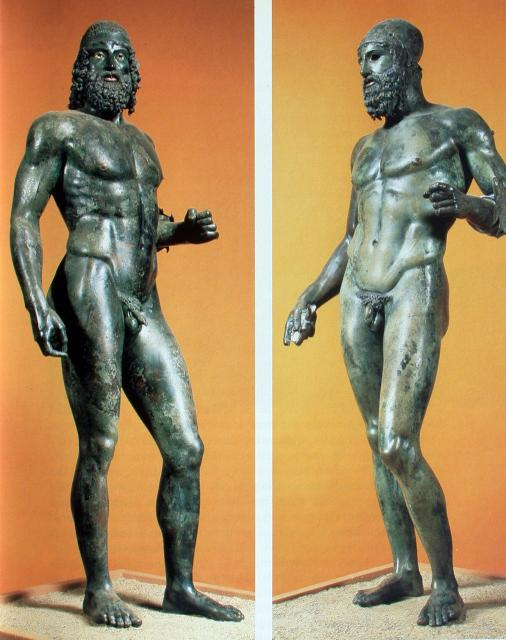Mr.Gold - Art 1 - Quiz #1
Quiz #1
Name - First , Last *
1) What does "Line Weight" refer to? *
5 points
2) This Hexagon is an example of a _______________ shape. *
3 points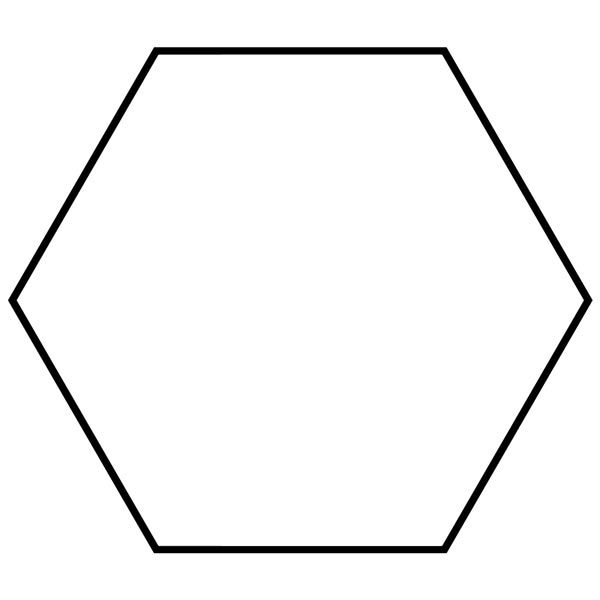3) These are examples of _________________ shapes. *
3 points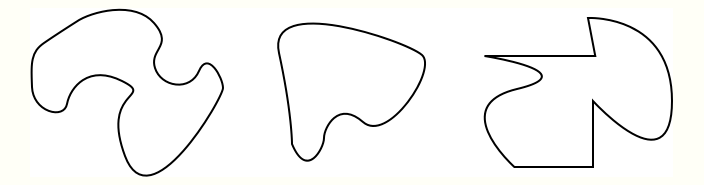4) Squares, triangles and circles are examples of shapes. Cylinders, spheres, and cubes are examples of _____________?
3 points
5) These are examples of _________________ shapes.
3 points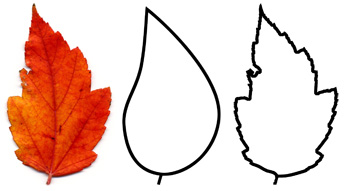6) What form of perspective is being utilized in this illustration? *
5 points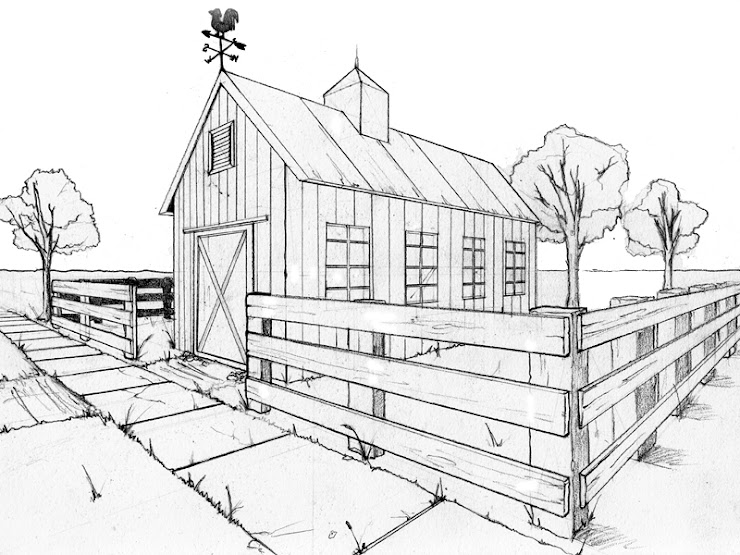7) What form of perspective is being utilized in this illustration? *
5 points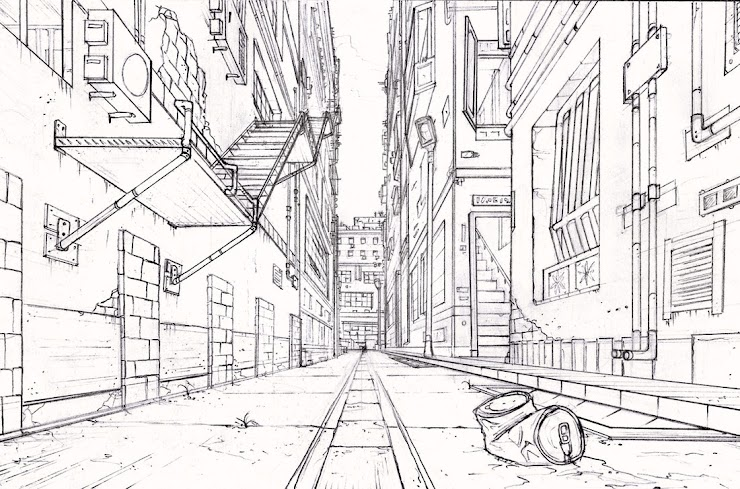8) What form of perspective is being utilized in this illustration? *
5 points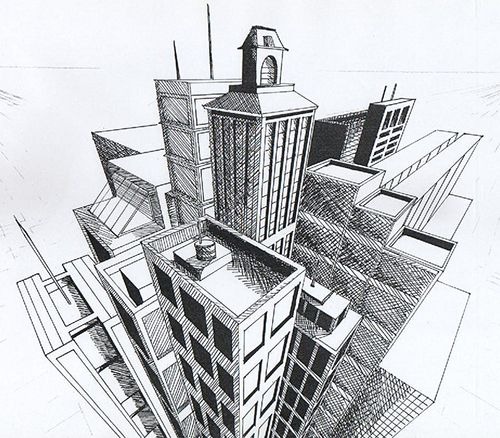9) The red lines in this 1-point perspective drawing are called what? *
5 points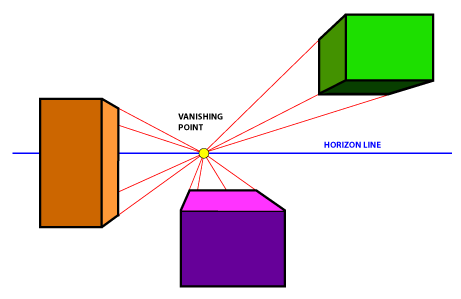10) In art, the ________________ (also called eye level) marks the point where the sky meets the land or water below. *
5 points
11) In linear perspective, the ___________________ is the spot on the horizon line to which the convergence lines meet. *
5 points
12) Two lines in a plane that do not intersect or touch each other at any point are called what...? *
4 points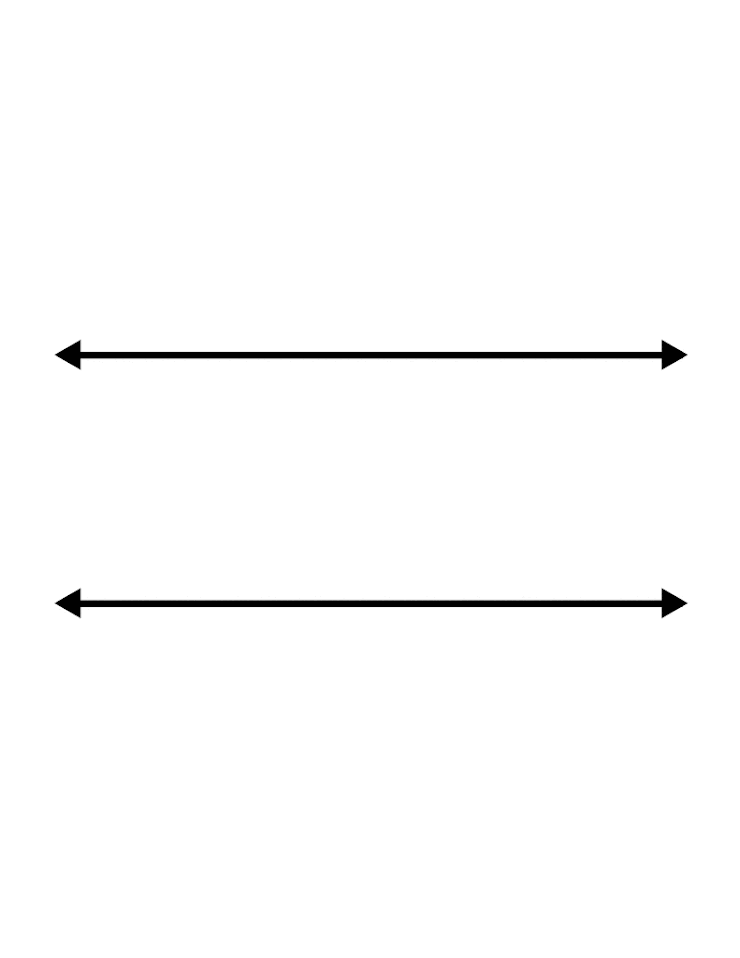13) Two lines that intersect at a 90 degree angle are ____________? *
4 points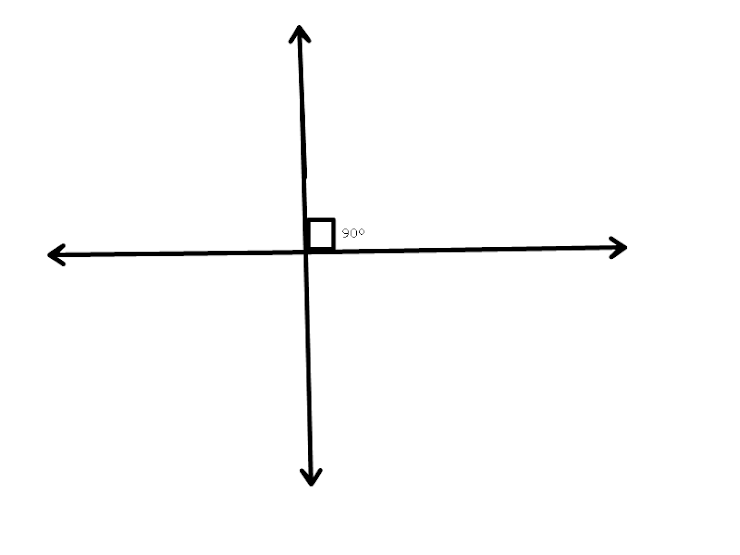14) What sort of shading technique is being used to add value to this sphere? *
5 points15) What sort of shading technique is being used to add value to this sphere? *
5 points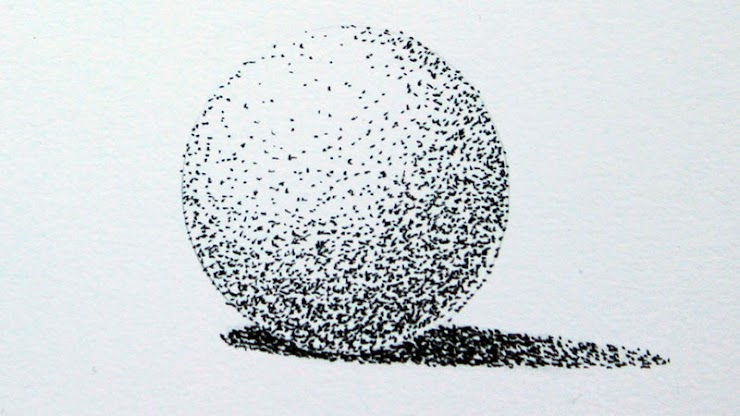16) What sort of shading technique is being used to add value to this sphere? *
5 points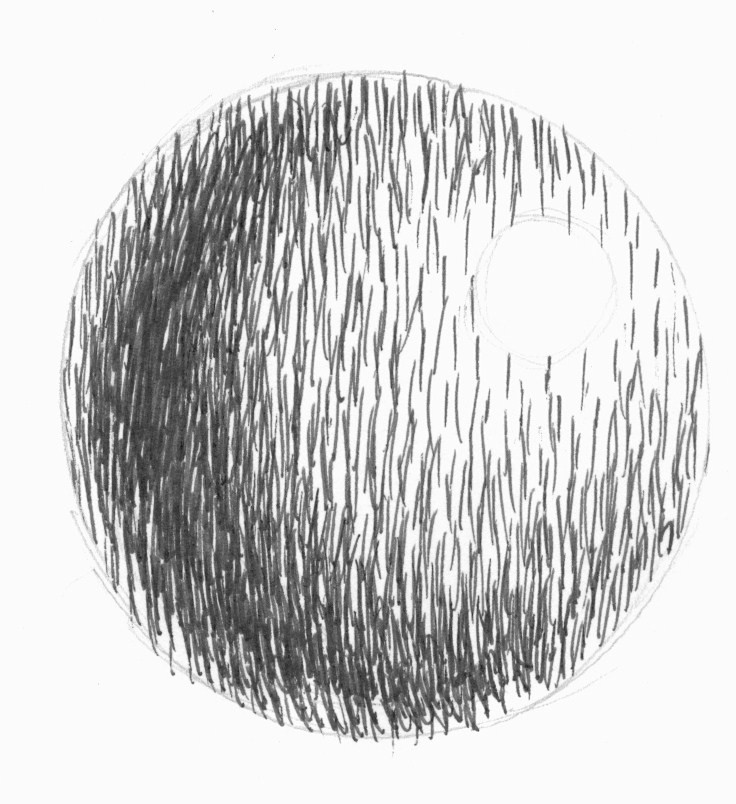17) What technique is being used in this drawing? *
5 points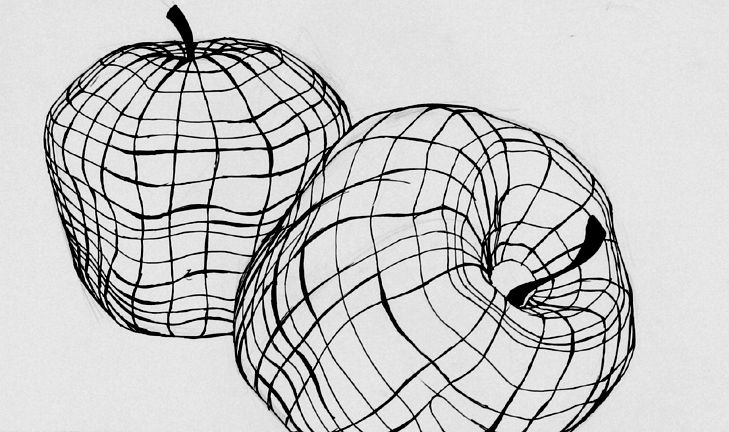18) What technique is being used in this drawing? *
5 points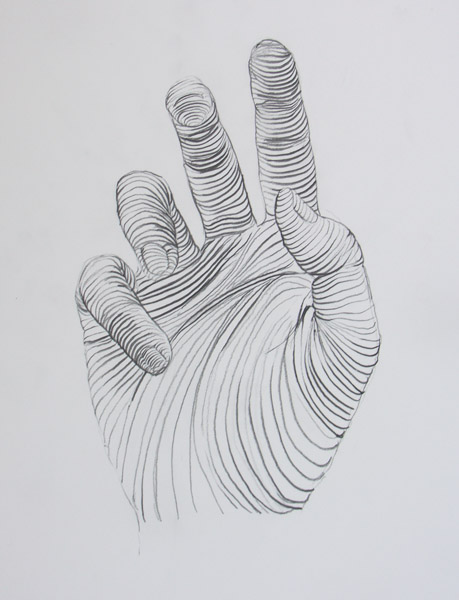19) What technique is being used in this drawing? *
5 points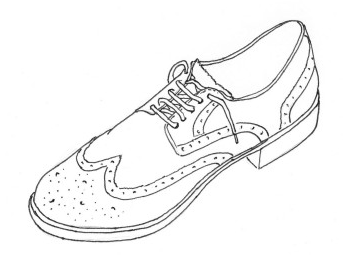20) What technique is being used in this drawing? *
5 points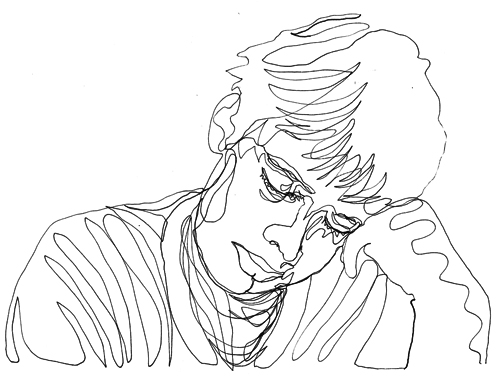21) What is the name of this 30,000 year old figurine? *
5 points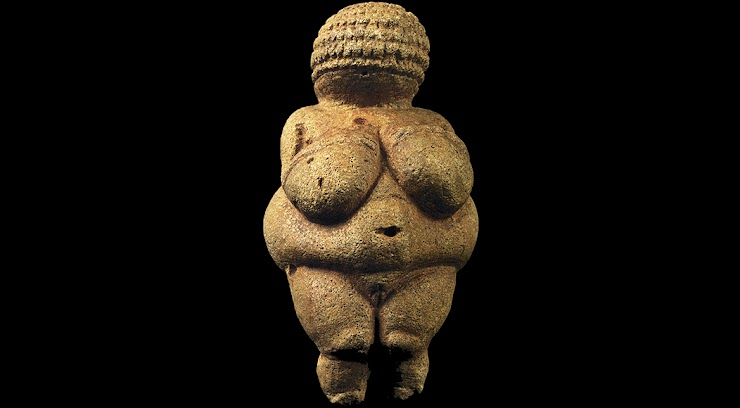What is the name of these famous bronze sculptures? *
5 points